DraftKings Championship Odds
+370
3.7 to 1Celtics
17.8% implied probability

+600
6 to 1Nets
11.9% implied probability

+800
8 to 1Bucks
9.3% implied probability

+800
8 to 1Nuggets
9.3% implied probability

+1000
10 to 1Warriors
7.6% implied probability

+1000
10 to 1Clippers
7.6% implied probability

+1100
11 to 1Grizzlies
7.0% implied probability

+1300
13 to 176ers
6.0% implied probability

+1900
19 to 1Suns
4.2% implied probability

+2200
22 to 1Cavaliers
3.6% implied probability

+2500
25 to 1Pelicans
3.2% implied probability

+3500
35 to 1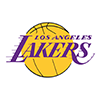Lakers
2.3% implied probability

+3500
35 to 1Mavericks
2.3% implied probability

+4000
40 to 1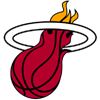Heat
2.0% implied probability

+7000
70 to 1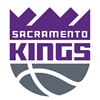Kings
1.2% implied probability

+9000
90 to 1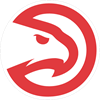Hawks
0.9% implied probability

+10000
100 to 1Raptors
0.8% implied probability

+12000
120 to 1Trail Blazers
0.7% implied probability

+20000
200 to 1Timberwolves
0.4% implied probability

+20000
200 to 1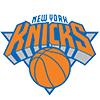Knicks
0.4% implied probability

+35000
350 to 1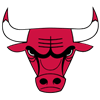Bulls
0.2% implied probability

+40000
400 to 1Jazz
0.2% implied probability

+50000
500 to 1Pacers
0.2% implied probability

+50000
500 to 1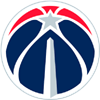Wizards
0.2% implied probability

+60000
600 to 1Thunder
0.1% implied probability

+100000
1000 to 1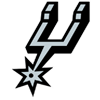Spurs
0.1% implied probability

+100000
1000 to 1Magic
0.1% implied probability

+100000
1000 to 1Hornets
0.1% implied probability

+100000
1000 to 1Rockets
0.1% implied probability

+100000
1000 to 1Pistons
0.1% implied probability